# Texas Go Math Grade 3 Lesson 11.3 Answer Key Even and Odd Numbers

Refer to our Texas Go Math Grade 3 Answer Key Pdf to score good marks in the exams. Test yourself by practicing the problems from Texas Go Math Grade 3 Lesson 11.3 Answer Key Even and Odd Numbers.

## Texas Go Math Grade 3 Lesson 11.3 Answer Key Even and Odd Numbers

Essential Question
How can you tell if a number is even or odd?
To tell whether a number is even or odd, look at the number in the ones place.
That single number will tell you whether the entire number is odd or even.
An even number ends in 0, 2, 4, 6, or 8. An odd number ends in 1, 3, 5, 7, or 9.

Unlock the Problem
Grace and her sister choose pairs of factors and guess if the products of their numbers are odd or even. What is the best guess for Grace—will most of the products be odd or even?

Remember
Even numbers end in 0, 2, 4, 6, or 8. Odd numbers end in 1, 3, 5, 7, or 9.

Activity
Find the product. Then determine if the product is even or odd.___ out of four possibilities in the table result in a product that is an ___ number. So, Grace should guess that most of the products will be ___.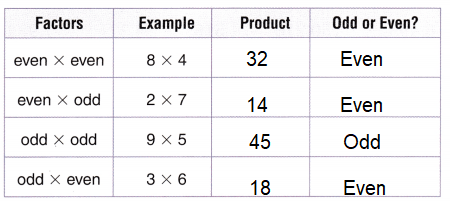Explanation:
A number which is divisible by 2 and generates a remainder of 0 is called an even number. An odd number is a number which is not divisible by 2.

• What if Grace chose 58 as a factor and her sister chose 67? Would the product be an odd or an even number? Explain your answer:
58 x 67 = 3886
Even x Odd = Even
Explanation:
A number which is divisible by 2 and generates a remainder of 0 is called an even number.
Try This! Tell if the product will be odd or even.
14 × 16
14 x 16 = 224     Even
Explanation:
A number which is divisible by 2 and generates a remainder of 0 is called an even number

231 × 7
231 x 7 = 1617      Odd
Explanation:
An odd number is a number which is not divisible by 2.

98 × 45
98 x 45 = 4410      Even
Explanation:
A number which is divisible by 2 and generates a remainder of 0 is called an even number

59 × 622
59 x 622 = 36698    Even
Explanation:
A number which is divisible by 2 and generates a remainder of 0 is called an even number

2,006 × 3
2006 x 3 = 6018   Even
Explanation:
A number which is divisible by 2 and generates a remainder of 0 is called an even number.

790 × 51
790 x 51 = 40290  Even
Explanation:
A number which is divisible by 2 and generates a remainder of 0 is called an even number.

Divisibility Rules
A number is divisible by another number if the number is a counting number and the number can be divided evenly. Some numbers have divisibility rules.

A number is divisible by 2 if the ones digit is 0, 2, 4, 6, or 8.
Divisible by 2:
64 98 412 7,940 80,576

Not divisible by 2:
63 97 411 7,939 80,575

• What do you notice about the numbers divisible by 2?
A number is divisible by 2,
if the given number is a must and should end with the 0, 2, 4, 6 and 8 number only.
If not ending with that numbers, the given number is Not divisible.
A number is divisible by 2 if the ones digit is 0, 2, 4, 6, or 8.

Try This! Circle the numbers divisible by 2.
445 867 3,428 6,499 150,210 516 4,321 7,934

Share and Show

Tell if the product will be odd or even.

Question 1.
even × odd
Explanation:
A number which is divisible by 2 and generates a remainder of 0 is called an even number

Question 2.
odd × odd
Explanation:
An odd number is a number which is not divisible by 2.

Question 3.
even × even
Explanation:
A number which is divisible by 2 and generates a remainder of 0 is called an even number.

Math Talk
Mathematical Processes

What conclusion can you draw when the product of two numbers is an odd number?

Circle the numbers divisible by 2.

Question 4.
47
Not divisible
Explanation:
In the given number 47,
7 is not ending with even number So,
the given number is not divisible by 2.
The given number should end with the 0, 2, 4, 6 and 8 number only, if not ending with that numbers, the given number is Not divisible by 2.
So, it is odd number.

Question 5.
598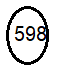Even
Explanation:
The given number 598, last number is ending with 8, 598 is divisible with 2.
A number is divisible by 2, the number should end with the 0, 2, 4, 6 and 8 number only. A number is divisible by 2 if the ones digit is 0, 2, 4, 6, or 8.

Question 6.
1,001
Not Divisible
Explanation:
A number is divisible by 2, if the given number ends with the 0, 2, 4, 6 and 8 number only. if not ending with that numbers, the given number is Not divisible.
so, the given number is Odd.

Question 7.
676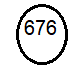Even
Explanation:
A number is divisible by 2, if the given number ends with the 0, 2, 4, 6 and 8 it is divisible.
A number is divisible by 2 if the ones digit is 0, 2, 4, 6, or 8.
So, the given number is Even.

Question 8.
3,794Even
Explanation:
A number is divisible by 2, if the given number is a must and should end with the 0, 2, 4, 6 and 8 number only. if not ending with that numbers, the given number is Not divisible
A number is divisible by 2 if the ones digit is 0, 2, 4, 6, or 8.

Use the divisibility rule to tell if the number is even or odd.

Question 9.
39
Not divisible by 2 and number is Odd.
Explanation:
The given number is  Not divisible by 2 as the last number is ending with 9 Odd,
and the given number 39 is Odd number.

Question 10.
472
Divisible by 2 and number is Even.
Explanation:
The given number is  divisible by 2 as the last number is ending with 2 even,
and the given number 472 is Even number.

Question 11.
2,500
Divisible by 2 and number is Even.
Explanation:
The given number is  divisible by 2 as the last number is ending with 0,
and the given number 2,500 is Even number.

Question 12.
934
Divisible by 2 and number is Even.
Explanation:
The given number is  divisible by 2 as the last number is ending with 4 even,
and the given number 934 is Even number.

Question 13.
6,281
Not divisible by 2 and number is Odd.
Explanation:
The given number is  Not divisible by 2 as the last number is ending with 1 Odd,
and the given number 6,281 is Odd number.

Question 14.
683
Not divisible by 2 and number is Odd.
Explanation:
The given number is  Not divisible by 2 as the last number is ending with 3 Odd,
and the given number 683 is Odd number.

Question 15.
1,748
Divisible by 2 and number is Even.
Explanation:
The given number is  divisible by 2 as the last number is ending with 4 even,
and the given number 1,748 is Even number.

Question 16.
20,645
Not divisible by 2 and number is Odd.
Explanation:
The given number is  Not divisible by 2 as the last number is ending with 1 Odd,
and the given number 20,645 is Odd number.

Question 17.
17,356
Divisible by 2 and number is Even.
Explanation:
The given number is  divisible by 2 as the last number is ending with 4 even,
and the given number 17,356 is Even number.

Question 18.
41,207
Not divisible by 2 and number is Odd.
Explanation:
The given number is  Not divisible by 2 as the last number is ending with 1 Odd,
and the given number 41,207 is Odd number.

Problem Solving

Question 19.
Olivia uses some beads to make 7 bracelets. She uses 5 beads on each bracelet. Will the number of beads Olivia uses be an even or an odd number? Explain.7 x 5 = 35
Explanation:
Olivia uses 35 beads to make 7 bracelets.
odd x odd = odd
7 and 5 are odd numbers and 35 is also odd number.

Question 20.
What’s the Error? Multi-Step Noah wrote the number 6,247. Bryson wrote the number 4,332. Both Noah and Bryson say their numbers are divisible by 2. Describe and correct the error.
No, only Noah number 6247 is divisible by 2.
Explanation:
Noah number 6247
Bryson number 4332
that last digit of the above number is ending with 2, so 4332 is divisible by 2 and 6247 is not divisible by 2

Question 21.
H.O.T. Multi-Step Vanessa is thinking of an even product between 40 and 50. One of the factors is even and one of the factors is odd. Both factors are 1-digit numbers. What is Vanessa’s number? Explain how you found it.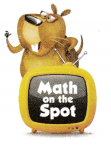Vanessa’s number is 42
Explanation:
Vanessa is thinking of an even product between 40 and 50, i.e., 42,44,46,48.
One of the factors is even and one of the factors is odd.
Both factors are 1-digit numbers.
So, 6 x 7 = 42.

Question 22.
H.O.T. Multi-Step The price tags on the two items Nathan wants to buy are 50¢ and 25¢. Will he pay for the two items with an even or an odd number of quarters? Explain.
Nathan pay odd number of 3 quarters.
Explanation:
The price tags on the two items Nathan wants to buy are 50¢ and 25¢.
Total price he has to pay 50¢ + 25¢ = 75¢
He pay for the two items with an an odd number of 3 quarters.
As, we know 1 quarter = 25¢.

Question 23.
Delia is playing an odd and even game with cards. She turns over a card and the number 25 shows. Which is true about 25?
(A) It is even because there is a 2 in the tens place.
(B) It is even because there is a 5 in the ones place.
(C) It is odd because there is a 2 in the tens place.
(D) It is odd because there is a 5 in the ones place.
Option(D)
Explanation:
Delia card turns over a card and the number 25 shows.
25 is odd number as 5 is present ones place.

Question 24.
Which of the following groups of numbers includes an odd number?
(A) 124, 556, 670
(B) 218, 844, 385
(C) 420, 774, 988
(D) 512, 230, 854
Option(B)
Explanation:
In the given numbers 218, 844, 385,
385 is odd number as 5 is present in ones place,
the rest of the numbers 218 and 844 the ones place has even number.
Question 25.
Multi-Step Jim is thinking of a mystery number between 50 and 70. His number is even and the sum of the digits is 9. What is Jim’s number?
(A) 45
(B) 72
(C) 54
(D) 63
Option(C)
Explanation:
Jim is thinking of a mystery number between 50 and 70.
His number is even and the sum of the digits is 9.
5 + 4 = 9
So, Jim’s number is 54.

Texas Test Prep

Question 26.
Which set of numbers contains all odd numbers?
(A) 238, 239, 240
(B) 591, 513, 510
(C) 423, 429, 431
(D) 156, 170, 192
Option(C)
Explanation:
The given numbers 423, 429, 431 contain odd numbers in ones place,
which are not divisible by 2.

### Texas Go Math Grade 3 Lesson 11.3 Homework and Practice Answer Key

Tell if the product will be odd or even.

Question 1.
37 × 41 ___
Explanation:
An odd number is a number which is not divisible by 2.
37 x 41 = 1517
odd x odd = odd

Question 2.
56 × 9 ____
Explanation:
A number which is divisible by 2 and generates a remainder of 0 is called an even number.
56 x 9 = 504
even x odd = even

Question 3.
64 × 88 ___
Explanation:
A number which is divisible by 2 and generates a remainder of 0 is called an even number.
64 x 88 = 5688
even x even = even

Question 4.
13 × 50 ____
Explanation:
A number which is divisible by 2 and generates a remainder of 0 is called an even number.
13 x 50 = 650

Circle the numbers divisible by 2.

Question 5.
113
Not divisible by 2
Explanation:
The given number is  divisible by 2 as the last number is ending with 3 even,
and the given number 113 is Even number.

Question 6.
572
Divisible by 2Explanation:
The given number is  divisible by 2 as the last number is ending with 2 even,
and the given number 572 is Even number.

Question 7.
488
Divisible by 2Even
Explanation:
The given number is  divisible by 2 as the last number is ending with 2 even,
and the given number 488 is Even number.

Question 8.
326
Divisible by 2Even

Explanation:
The given number is  divisible by 2 as the last number is ending with 2 even,
and the given number 488 is Even number.

Question 9.
631
Not divisible by 2
Explanation:
The given number is  divisible by 2 as the last number is ending with 3 even,
and the given number 113 is Even number.
Question 10.
234
Divisible by 2Even
Explanation:
The given number is  divisible by 2 as the last number is ending with 2 even,
and the given number 488 is Even number.

Problem Solving

Question 11.
Kenji writes the numbers 4,230 and 5,607. He says that both numbers are divisible by 2. Is Kenji correct? Explain.
No, he is not correct.
Only one number 4,230 is even.
Explanation:
Kenji writes the numbers 4,230 and 5,607.
4,230 is only divisible by 2 as 0 is present in ones place.
But in 5,607 odd number 7 is present in ones place, which is not divisible by 2.
No, Kenji only number 4,230 is even.

Question 12.
Kara uses some buttons to decorate 6 puppets. She uses 8 buttons on each puppet. Will the number of buttons Kara uses be an even or an odd number? Explain.
48 buttons
Explanation:
Kara uses some buttons to decorate 6 puppets.
She uses 8 buttons on each puppet.
6 x 8 = 48
The number of buttons Kara uses are even.

Question 13.
Miguel has a group of 17 red counters and a group of 13 yellow counters. He arranges the counters into stacks of 5. Does he have an odd or even number of stacks? Explain.
6 stacks
Explanation:
Miguel has a group of 17 red counters and a group of 13 yellow counters.
Total counter 17 + 13 = 30
He arranges the counters into stacks of 5.
30 ÷ 5 = 6
Miguel have an even number of stacks.

Texas test Prep

Lesson Check

Question 14.
Harry tosses three number cubes to make this number.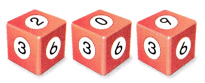Which is true about Harry’s number?
(A) It is even because there is a 2 in the hundreds place.
(B) It is even because there is a 0 in the tens place.
(C) It is odd because there is a 9 in the ones place.
(D) It is even because is it divisible by 2.
Option(C)
Explanation:
It is odd because there is a 9 in the ones place, which is not divisible by 2.

Question 15.
Dale tosses three number cubes shown below.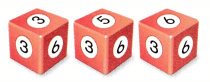Which is an even number Dale can make from the numbers?
(A) 536
(B) 635
(C) 563
(D) 365
Option(A)
Explanation:
536 is even number, because it is exactly divided by 2 and 6 is present in ones place.

Question 16.
Gerard tosses four number cubes shown below.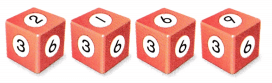Which set of numbers that Gerard makes are all odd?
(A) 216, 619, 926
(B) 169, 921, 269
(C) 692, 961, 219
(D) 926, 261, 692
Option(B)
Explanation:
In the above numbers 169, 921, 269 all ends with odd numbers in ones place,
which are not divisible by 2.

Question 17.
Multi-Step A mystery number is odd and has 2 digits. The sum of the digits is 11. The digit in the tens place is between 0 and 3. What is the mystery number?
(A) 29
(B) 38
(C) 47
(D) 56
Option(A)
Explanation:
A mystery number is odd and has 2 digits.
The sum of the digits is 11.
1 + 1 = 2
The digit in the tens place is between 0 and 3, i.e., 2.
So, the mystery number is 29.

Question 18.
Multi-Step A mystery number is even and has 2 digits. The difference between the digits is 3. The digit in the ones place is between 0 and 4. What is the mystery number?
(A) 30
(B) 63
(C) 74
(D) 52